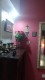# Find Index of Maximum & Minimum Value of Vector & Data Frame Row in R (2 Examples)

In this article you’ll learn how to identify the index of maxima and minima of vectors and data frames in the R programming language.

The tutorial will consist of this information:

Let’s dive into it…

## Example 1: Determine Index of Maximum & Minimum of Vector

Example 1 explains how to find the max and min value of a numeric vector in R. First, we need to create an example vector:

`vec <- c(3, 1, 8, 3, 2, 5)                     # Create example vector`

Our example vector consists of six numeric vector elements. Now, we can apply the which.max function to find the index of the maximum value…

```which.max(vec)                                 # Identify index of max value
# 3```

…and the which.min function to determine the index of the minimum:

```which.min(vec)                                 # Identify index of min value
# 2```

As you can see based on the previous outputs of the RStudio console, the max value is at position 3 and the min value is located at position 2 of our example vector.

## Example 2: Determine Index of Maximum & Minimum of Data Frame Column

We can use a similar R syntax as in Example 1 to determine the row index of the max or min value of a data frame column. First, we have to create some example data:

```data <- data.frame(x1 = c(7, 8, 1, 4, 0, 5),   # Create example data frame
x2 = letters[1:6])
data                                           # Print data to RStudio console
#   x1 x2
# 1  7  a
# 2  8  b
# 3  1  c
# 4  4  d
# 5  0  e
# 6  5  f```

Our data matrix contains two columns and six rows. Let’s assume that we want to find the index of the rows with the min and max values of our variable x1. Then, we can apply the which.max…

```which.max(data\$x1)                             # Apply which.max function to column
# 2```

…and which.min functions:

```which.min(data\$x1)                             # Apply which.min function to column
# 5```

The highest value of the column x1 is located in row 2 and the lowest value of the column x1 is located in row 5.

## Video, Further Resources & Summary

If you need more information on the R programming code of this post, you may have a look at the following video of my YouTube channel. I illustrate the R programming code of this page in the video:

Please accept YouTube cookies to play this video. By accepting you will be accessing content from YouTube, a service provided by an external third party.If you accept this notice, your choice will be saved and the page will refresh.

Furthermore, I can recommend to read the related tutorials of this website. Please find a selection of articles here.

In summary: At this point you should know how to get row-wise minima and maxima in the R programming language. If you have any further questions, let me know in the comments.

Subscribe to the Statistics Globe Newsletter

•November 12, 2021 2:45 am

if the minimum value in at 2 places then what is the syntax

•Neena
August 27, 2022 1:06 pm

Hi. I have two columns. One consisting of the names of story books and novels and another consisting of their ratings. How do I find the name of the novel with the lowest rating?

•August 29, 2022 9:38 am

Hey Neena,

Please have a look at the following example:

```data <- data.frame(books = c("book_a", "book_b", "book_c"),
ratings = c(2, 1, 3))
data
#    books ratings
# 1 book_a       2
# 2 book_b       1
# 3 book_c       3

data\$books[which.min(data\$ratings)]
#  "book_b"```

Regards,
Joachim

•Neena
August 29, 2022 11:20 am

Hi Joachim.

This works well for me. Thank you.

Regards

Neena

•August 29, 2022 11:37 am

Hi Neena,

That’s great to hear, glad it helped! 🙂

Regards,
Joachim

•Jack
October 25, 2022 10:51 pm

Hi, If we have three columns
data <- data.frame(number = c(4,6,7),
ratings = c(3, 4, 6),
sales=c(1,1,7)
)
data

How do I find the name of the variable with the min, and how many values in that variable are smaller than the other two variables? which is here sales and 2 values smaller.

•November 14, 2022 12:43 pm